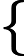Study of mathematics online.
Study math with us and make sure that "Mathematics is easy!"

# System of linear equations - matrix form

Any system of linear equations can be written as the matrix equation.

So a system of linear equationsa11x1 + a12x2 + ... + a1nxn = b1 a21x1 + a22x2 + ... + a2nxn = b2 ································ am1x1 + am2x2 + ... + amnxn = bm

consisting of m linear equations with n unknowns can be written as a matrix equation:

Ax = b

where

 A =a11 a12 ... a1n;     x =x1;     b =b1a21 a22 ... a2n x2 b2 ······························ ··· ··· am1 am2 ... amn xn bm

Matrix A is the matrix of coefficient of a system of linear equations, the column vector x is vector of unknowns variables, and the column vector b is vector of a system of linear equations values.

N.B. If the i-th row of the system of linear equations is not the variable xj, it means that it multiplier is zero, ie aij = 0.

## Example of matrix form of system of linear equations

Example 1.
Write system of linear equations in matrix form:4x1 + x2 - x3 - x4 = 3 -x1 + 3x3 - 2x4 = 5 6x1 + 2x2 + 4x3 = 2 2x2 - x3 + x4 = 0

Solution: System of linear equations in matrix form:4 1 -1 -1·x1=3-1 0 3 -2 x2 5 6 2 4 0 x3 2 0 2 -1 1 x4 0